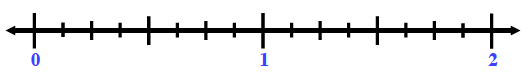### Home > ACC6 > Chapter 6 Unit 6 > Lesson CC1: 6.2.2 > Problem6-88

6-88.

Draw a number line from $0$ to $2$. Then write the following numbers in its correct place on the number line.

$0.2$          $\frac { 13 } { 26 }$          $1.5$          $\frac { 1 } { 8 }$          $1.9$          $\frac { 7 } { 8 }$          $1.09$          $\frac { 3 } { 5 }$          $1.19$Decimal to fraction:
Use the digits as the numerator. use the decimal place value as the denominator. Simplify as needed.
$0.48=\frac{48}{100}=\frac{12}{25}$

Fraction to decimal:
Divide the numerator by the denominator.
$\frac{3}{8}=3\div8=0.375$